# Rotational Puzzles on Graphs

On this page I will discuss various graph puzzles, or rather, permutation puzzles consisting of partially overlapping cycles. This was first investigated by R.M. Wilson in his paper titled 'Graph Puzzles, Homotopy, and the Alternating Group' [J. Combin. Theory (Series B) 16 (1974) 86-96.] Most of what I write below has parallels in that paper, though I solve a subtly different problem, and decided to do it in my own way.

You will need to have some knowledge of permutations and permutation parity to understand this page, and to understand the proofs some elementary knowledge of permutation groups and group theory is needed.

## Graph puzzles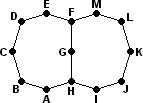Suppose we have some graph, for example the one shown on the right. That is to say, there are several points (called nodes or vertices) in the flat plane, and there are lines (called edges) connecting pairs of these points. (See the Cayley Graphs page for a more detailed explanation.) Suppose further that the graph is connected, that every node has at least two edges, and that there are no loops (i.e. no edges that connect a node to itself). This is the case for the example graph on the right. There are now two ways of turning such a graph into a permutation puzzle.

### Sliding type of graph puzzle: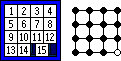A graph can be turned into a slide puzzle. In this case pieces are placed on all the nodes, except that one node is left empty. A move consists of sliding a piece along an edge to the empty node. For example, the standard Fifteen puzzle is based on a 4x4 grid graph, as shown on the right, with the corner node initially empty.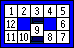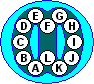The example graph could be turned into a sliding piece puzzle looking much like the Nineball puzzle. It can also be done with square tiles, as shown on the far right. Of course, most graphs cannot be realised with square tiles, though using beads or balls inside tracks will often work.

### Rotational type of graph puzzle: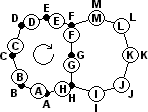A graph can be turned into a rotational puzzle by putting a puzzle piece on every node, not leaving any nodes empty. The graph has several faces - in the case of this example there are two faces, one on the left and one on the right, both having 8 nodes around them. A move consists of shifting along all the pieces around a face clockwise (or anti-clockwise) to the next node.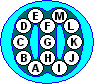The example graph would result in a physical puzzle like the one shown on the right. It has two looped tracks containing 8 beads, and the two loops share 3 beads between them.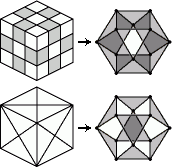Note that we assume the puzzle has only one type of piece. The Rubik's Cube for example has edges and corners. The positioning of these can be analysed separately with this theory, but positioning them all at the same time is not dealt with. On the right you see how the edges of the Rubik's cube form a graph puzzle. Also shown is the graph for the Dino cube. Note that the graphs are exactly the same, except that different faces are allowed to move. The Rubik's graph only allows the square faces to move, the Dino graph only the triangular faces.

The sliding type of puzzle is very similar to the rotational type. Suppose you slide the pieces so that the blank space goes around one face, returning to its starting spot. This has the effect of the pieces in that face, just as if it was a rotational puzzle without the empty node. If the blank is not in the face you want to turn, you can bring it to the face, go around it, and take the same route back. This cycles around all but one of the pieces in that face. This is subtly different from the rotational puzzle, where all the pieces in a face take part in a turn. The methods I use for rotational puzzles will also apply with minor adjustments to the sliding puzzles. Richard M. Wilson's paper dealt exclusively, and exhaustively with the sliding puzzles, so I will only examine the rotational type of puzzle on the rest of this page.

## Even and odd parities

Suppose we have a graph in which all the faces have an odd number of nodes. Every move in our puzzle is then a cycle involving an odd number of pieces, and such cycles have even parity. In this kind of graph puzzle, clearly no odd permutations are possible. The best we can hope to do is to be able to perform any even permutation. As we shall see later, in most graphs all even permutations can indeed be achieved.

Suppose instead that we have a graph that contains at least one face with an even number of pieces, i.e. there is some move that is an odd permutation. If we can prove that all even permutations are possible, then by using that odd move we can also get all odd permutations. In other words, every permutation becomes possible, regardless of parity. The proof follows:

Parity Lemma: Let An be the alternating group of n items, i.e. it is the group of all even permutations of n items. Let p be some permutation (of some or all of those n items) that has odd parity. Let G=<An,p>, i.e. let G be the group generated by the elements of An and p. Then G=Sn.

Proof: Let q be any element in Sn. If q is an even permutation, then it is in An and hence in G=<An,p>. If on the other hand q is an odd permutation, then r=qp is an even permutation. Since r then lies in An and thus in G, so does q=rp-1. Therefore G contains q regardless of its parity, i.e. G contains the whole of Sn. But G is a subgroup of Sn so it must actually be equal.

What remains now is to find a way to use the moves to produce all the even permutations.

## From large to small

When playing with permutation puzzles, you may have noticed that the more faces a puzzle has, the easier it is to solve some part of it. You have more degrees of freedom, and you can use the unsolved area of the puzzle to move the pieces about the way you want them. It is only at the end, when you have only one or two unsolved faces that it becomes really hard. Conversely, if you can solve those last one or two faces, then you are surely accomplished enough to be able to solve the whole puzzle.

The same holds for these graph puzzles a well. If you can solve those last two faces completely without using the other faces, then you can use that knowledge to solve the whole puzzle. This can be stated more formally as follows:

Extension Lemma: Let An be the alternating group of n items (n>2), i.e. it is the group of all even permutations of n items. Let c be the cycle (k k+1 k+2 ... m), where k≤n<m. Then <An,c> contains Am.

In order to prove this, we need the following lemma:

Lemma: An is generated by the 3-cycles (1 2 x) for x=3..n.

Proof: Let Tn={(1 2 x) | x=3..n} be the set of supposed generators of An. We need to show that An=<Tn>.
It is obviously true when n=3. For larger n it can be proved by induction.
Suppose the lemma holds for some value of n. Let p be any permutation in An+1. Suppose p sends item n+1 to some item k. Then q = p (1 2 k) (1 2 n+1)2 will send n+1 back to itself. In other words, q can be considered an even permutation of items 1 to n only, and as such, it lies in An=<Tn> and hence certainly lies in <Tn+1>. Therefore, p = q (1 2 n+1) (1 2 k)2 will also lie in <Tn+1>. This shows that An+1 is a subset of <Tn+1>, and it is easy to see they must be equal. By induction the lemma therefore holds for all values of n>2.

Now we can prove the Extension Lemma:

Proof of Extension Lemma: Let's first assume that k>2.
Using conjugation of (1 2 n) with c, we find that:
c-i (1 2 n) ci = (1 2 n+i), for any 0≤i≤m-n.
This shows that <An,c> contains all 3-cycles (1 2 x) with x=3..m. Therefore it must contain Am.
Suppose now that k=2, i.e. c is the cycle (2 3 4 ... m). Then
(1 2 3) c-i (1 3 2) ci (1 2 3) c-i (1 2 3) ci (1 3 2) =
(1 2 3) (1 3+i 2+i ) (1 2 3) (1 2+i 3+i ) (1 3 2) = (1 2 2+i) for i>1.
Therefore we again have all 3-cycles of the form (1 2 x) with x=3..m, so <An,c> contains Am.
Finally, suppose now that k=1, i.e. c is the cycle (1 2 3 ... m). Then let d = c(1 3 2) = (3 4 ... m). By applying the first case above to d, we find that <An,c>=<An,d> contains Am.

In other words, if we can use just a few faces and get every even permutation of their pieces, then we can add another adjacent face and get every even permutation of this larger set of pieces. By adding the faces one by one, we can then get every even permutation of the whole puzzle.

Note that in the formulation of this lemma I have assumed that the added face only has one area of overlap with the rest. This is hardly a restriction however. If you can do every even permutation on a set of pieces, then you can certainly do every even permutation on a subset of those pieces. You can therefore just remove the extra overlaps from the set so that your set and the added face have only one overlap. As long as the set still has at least three pieces, it will still work.

From the above it is clear that we need to examine the smallest cases first, i.e. the graphs involving only two faces. If those two faces allow for all even permutations, then the Extension Lemma shows the whole puzzle allows all even permutations.

## Two-faced puzzles

I will now assume that we only have two faces that share only one section. Most rotational puzzles have a pair of faces like that. If however every pair of intersecting faces on your puzzle has 2 or more overlaps, then the theory below does not apply. I will return to this point later on.

Any rotational graph puzzle with two faces that have one shared section can be specified using 3 numbers:
- the number of pieces unique to the first face
- the number of pieces shared by the two faces
- the number of pieces unique to the second face
I will use the notation (x,y,z) to specify the puzzle with y pieces shared between the two faces, x and z pieces unique to the two faces.

In the sections below, this general (x,y,z) problem is split into many separate cases which are mostly handled in very similar ways, though there are differences in the details. Each time we will establish a 3-cycle, preferably of three adjacent pieces. Applying the Extension Lemma to the combination of that 3-cycle (or actually A3) and a turn of one affected face, it follows that all even permutations in that face can be achieved. Then another application of the Extension Lemma shows that the two faces together allow every even permutation of the whole set of pieces.

The cases we will treat like this are:

At the very end there are two unusual special cases which do not allow all even permutations:

## Case (1+,1,1+)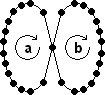Let's start with the simplest case - the two faces have only one piece in common. Call the two faces a and b. The same two letters are also used for clockwise moves of those faces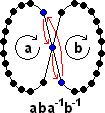As you may have expected with moves that have little overlap, commutation is a fruitful technique. The commutator aba-1b-1 of a and b is a 3-cycle, as illustrated here.

As mentioned before, we can apply the Extension Lemma to show that the 3-cycle and a turn of one face itself combine to make every even permutation of the pieces in that face possible. Applying the Extension Lemma once more shows that in the two faces combined, any even permutation of the whole set of pieces can be achieved.

There are many examples of this type of puzzle. For example, the Pyraminx and the Dino Cube are (2,1,2) puzzles, the Octahedron is a (3,1,3) puzzle, and the Alexander's Star is a (4,1,4) puzzle. The 'edge' pieces of the Turnstile form a (5,1,5) puzzle.

## Case (2+,2+,3+)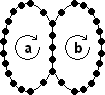Let's move on to the more complicated case where the two faces have several adjacent pieces in common.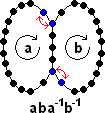Again a commutator is useful, but this time aba-1b-1 does not give a 3-cycle. Instead it does a double swap: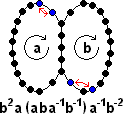We can now conjugate this in order to make face a be affected by only a single swap, the other swap having been parked out of the way in the unshared part of face b. The move sequence b2a(aba-1b-1)a-1b-2 does this. Face a now has only one adjacent swap in it: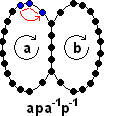Let's call the previous sequence p. Commutating this with a, we get a 3-cycle in face a which doesn't affect any pieces from face b at all.

Now that we have this 3-cycle, the same reasoning as before shows every permutation of the whole puzzle is possible. Unfortunately, there is a tacit assumption in the above proof. It is assumed that face b has at least 3 pieces that are not part of face a, and that face a has at least 2 pieces not part of face b. Of course we can turn the puzzle around and apply the same moves with the faces a and b swapped. Therefore the only cases left are when a and b both have exactly 2 unshared pieces, the case where one or both faces only have a single unshared piece, and the case where one face has no unshared piece at all.

An example of a puzzle of this type is the Impossiball. Any pair of adjacent faces is a (3,2,3) puzzle.

## Case (0,2+,1+)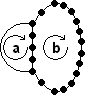In this case all the pieces of one face are also part of the other face. I doubt there will ever be a mechanical puzzle that has this configuration with more than 2 shared pieces, but it has to be included here for completeness.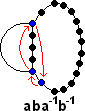At first sight it is surprising that the commutator is so simple - aba-1b-1 is simply a 3-cycle: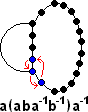It is easy to conjugate this by a to get a 3-cycle of adjacent pieces a(aba-1b-1)a-1:

Obviously this assumes that face b has at least one unshared piece. If it hadn't, then the two faces would have the same effect, and there would really only be one face.

## Case (1,2+,1)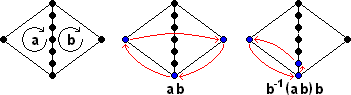First we'll see what we get when the faces each have a single unshared piece. This turns out to be a fairly easy case. The move sequence ab is a 3-cycle. It is easy to conjugate it into a 3-cycle in only one face, namely b-1(ab)b:

## Case (1,2,2+)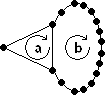Next we'll see what we get when only one of the faces, say face a, has a single unshared piece. If the face has 3 pieces (two shared with b) it is very simple. A move of face a already is a 3-cycle, so all even permutations of its 3 pieces are trivially possible. We can immediately apply the Extension Lemma to get every even permutation of the whole puzzle.

This reasoning actually works for the (1,2,1) case as well, though that was already handled in the previous case. An example of a puzzle of this type is the Skewb Diamond. The corners of any pair of adjacent faces form a (1,2,1) puzzle.

## Case (1,3,3+)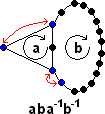Suppose there are exactly 3 shared pieces. As with the (2+,2+,3+) case, the effect of the commutator is two swaps.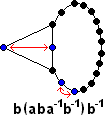The pieces can't be parked in quite the same way as before, but if face b has at least 3 unshared pieces, it is still not too hard to create a 3-cycle. Conjugating the commutator with b, we get b (aba-1b-1) b-1, which does one swap in face a, and the other swap in face b's unshared pieces.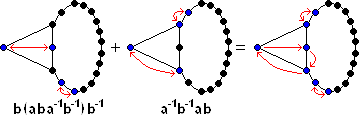Combining that with the commutator a-1b-1ab we get a 3-cycle and two swaps:

Doing that permutation twice, the two swaps disappear, leaving a pure 3-cycle in face a. As before, this is enough to show that every even permutation can be achieved. We temporarily disturbed three of the unshared pieces of face b while constructing our 3-cycle. This method therefore does not work if b has only 2 unshared pieces. The special case (1,3,2) is still open, but it will be dealt with later.

## Case (1,4+,2+)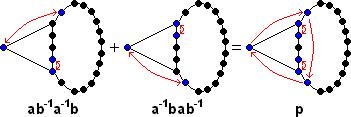Finally suppose there are 4 or more pieces shared amongst the two faces. We can combine the commutator ab-1a-1b and its mirror image a-1bab-1 to get a 3-cycle and two swaps: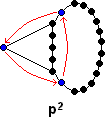Applying this permutation twice, the swaps disappear, leaving only a 3-cycle: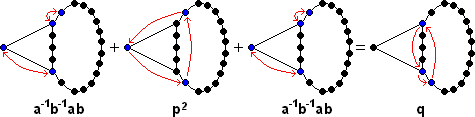At this point we might be able to solve the puzzle already, but I would prefer to have a more useful 3-cycle, consisting of 3 adjacent pieces in one face. Conjugation by a-1b-1ab gives the following 3-cycle: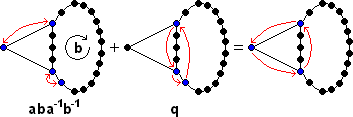Pre-multiplying it by aba-1b-1 gives a 3-cycle of adjacent pieces in face a. As before, this is enough to show that every even permutation can be achieved.

## Case (2,3+,2)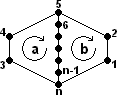This is by far the trickiest case so far. If we label the locations 1 to n as in the picture on the right, then:
a = (3 4 5 ... n-1 n)
b = (n n-1 ... 5 2 1)
c = a'bab' = (1 3)(5 6)
d = a'b'ab = (2 5)(3 n)
e = aba'b' = (1 n)(4 5)
f = a' d a = (2 6)(3 4)
g = c' d c = (2 6)(1 n)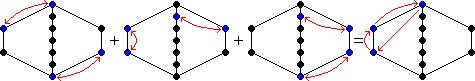By combining those various double swaps, we get a 3-cycle in one face:
efg = (4 5)(3 4) = (3 4 5)

## Case (2,2,2)

The only two-faced graphs that don't fall under one of the previous cases are (2,2,2) and (1,3,2). Up till now, every graph we encountered allowed all even permutations to be performed on its pieces. The (2,2,2) graph is different however. Only 5!=120 positions can be achieved instead of the 6!=720 that you would normally expect when permuting six pieces. This case is so unusual, and the proof quite tricky, that I have put it on a separate web page about the Two-generator corners group.

## Case (1,3,2)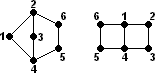Label the 6 pieces in the (1,3,2) puzzle as in the picture on the right. Its moves are then a=(1234), b=(65432). Similarly, label the 6 pieces of a (2,2,2) puzzle as shown, and then its moves are A=(1234), B=(1456).

Note that B = b-1a-1, A=a, and conversely, a=A, b=A-1B-1. Every move sequence on one of these graphs can thus be converted and performed on the other. This proves (1,3,2) puzzle has exactly the same set of positions as the (2,2,2).

## Extending the special cases

The two special cases (2,2,2) and (1,3,2) are the only ones where not all even permutations can be achieved. If a third face is added to these two, can every even permutation be achieved then? If the third face and one of the other two form one of the previously described non-special puzzles, then every even permutation is possible. Therefore the third face must form a special case (2,2,2) or (1,3,2) with any face it intersects with.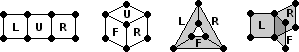This leaves only very few possibilities. Suppose we extend the (2,2,2) case with another face that has 4 nodes. The only ways to do it so that any overlapping pair of faces form a (2,2,2) puzzle are shown on the right. The first case may be familiar to you on the Rubik's Cube. There is a move sequence LU'R'UL'U'RU which is a 3-cycle of corners in the U face. This establishes that, as usual, all even permutations are possible in the first graph. Since a 4-cycle is an odd permutation, the Parity Lemma shows that all permutations, odd and even, are possible. The second graph puzzle is just like positioning the corners of a 2×2×2 cube, and the third graph corresponds to the Roundy puzzle. In both of these and in the fourth graph all permutations are possible as well.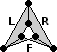The third graph above, of the Roundy puzzle, has one more face than the (2,2,2) graph but still has only 6 nodes. The added face just connects up the two sides of the (2,2,2) graph to form a triangular cylinder. It is also possible to put them together with a twist, forming a Möbius strip. This does not introduce any new positions however, since then L2 R L2 is a turn of that face. There are many other 4-cycles in the group, all of which could be added to the graph as a face without changing the number of puzzle positions. Any added face that introduces new nodes to the graph will however allow all permutations to be generated.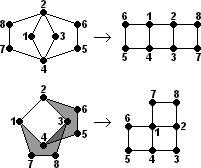Suppose we extend the (1,3,2) case with a new face that adds at least one node to the graph. Whichever way we extend it with a square face, there will always be two faces that form a puzzle of the type (3,1,3), (3,1,4), (1,3,1), or (2,2,3). If we extend it by a face with 5 nodes, the only ways that don't obviously give all even permutations are shown on the right. The added face forms a (1,3,2) puzzle with the existing square face.
Using the correspondence noted in the (1,3,2) section, these graphs puzzles are found to be equivalent to ones with only square faces. These have already been shown to allow all permutations.

## ConclusionNearly every permutation puzzle with two or more rotating faces allows every even permutation to be achieved. Furthermore, if any face contains an even number of pieces, then all odd permutations are also possible.
There are trivial exceptions to this rule, such as puzzles with only one face, but the only non-trivial exceptions are the two puzzles, both with exactly six pieces, shown on the right. These two puzzles have only 5! positions instead of the 6! positions you would normally expect.

## Comparison to sliding piece puzzles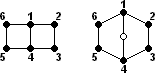In Richard M. Wilson's paper the sliding piece puzzles on general graphs were analysed. He came to a similar conclusion as above, namely that nearly every sliding piece puzzle on a graph allows all even permutations, and if there is any cycle with an odd number of nodes then all odd permutations are possible too. There are trivial exceptions of course, namely graphs that just consist of a single cycle, and also separable graphs, i.e. graphs where removal of a single node can split it into disconnected parts. The only non-trivial exception he found is the graph shown on the far right, which has only 5! permutations with the blank in the centre. That graph neatly corresponds to one of the exceptional graphs I found for the rotational puzzles, namely the (2,2,2) graph shown on the near right. Wilson's graph is the same except that it has an extra node in the middle that holds the blank space.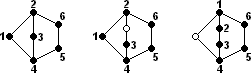The graph on the near right is second exceptional graph I found, namely the (1,3,2) graph. Next to it is the corresponding sliding puzzle graph, where a node has been added in the middle to hold the blank space. A closer look however reveals that it is in fact exactly the same as Wilson's graph, except that the blank is at a different place.

When discussing two-faced puzzle, I assumed that the faces have only one section in common. There are puzzles however which don't any pair of faces like that, i.e. every pair of intersecting faces have two or more overlapping sections. For example, puzzles such as the Rubik's Rings / Hungarian Rings are not covered by the theory as outlined here. The reason is that its (planar) graph has three faces, but only two can be moved in the real puzzle. Furthermore, those two faces overlap in two places rather than one. If it were a sliding puzzle however, then the blank could go around the middle face as well and so allow any even permutation. For the theory of generalised Hungarian Rings, see Singmaster's Cubic Circular 5/6, p9-10.
Another example of such a puzzle is the Equator / Hungarian Globe. In this puzzle we clearly cannot get every even permutation since pairs of opposite tiles will always remain opposite.

After all this, there remains lots of unexplored territory. Here are some things that you may want to look into yourself.

• What happens if the faces have centres with a visible orientation? In most cases I used commutators and conjugations, which don't affect face centre orientations. Then the centres can be solved first before doing the pieces. However case (1,2+,1) in particular was not done that way.
• What happens if there are several independent sets of pieces on each face, e.g. just like corners and edges on the cube? You would have a different graph for each type of piece, and the corresponding faces of the graphs would usually have the same number of nodes, just a different amount of overlap with the neighbouring faces.
• What can happen if all adjoining faces have more than one overlap? I already gave two examples above, but another neat case is given by the three moves/faces (1 2 6 5), (1 3 6 4), and (2 3 5 4) which generate only 24 positions (hint: think rolling die).
• What happens if a move is not a single cycle? Examples include the Topspin, or the corners of the Rubik's Domino, as well as many others. Many of them involve 180 degree turns, giving lots of 2-cycles.